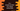# How to access an element of a vector using index in C++## How to access an element of a vector using index in C++:

We can access a vector element in different ways. Vectors are dynamic in nature, i.e. if we add an element to a vector, it increases its size by 1. But, we can access an element using the index of that element. In this post, we will learn how to access an element of a C++ vector.

### Index of vector elements:

Each elements of a vector can be accessed by using its index. It works similar to array, i.e. the index of the first element is 0, index of the second element is 1 etc.

We can access an element in two different ways:

1. By using the [] operator and
2. By using at() method.

Let’s check how to do that with example for both.

### Method 1: By using the [] operator:

[] operator is defined as like below:

``reference operator[] (size_type n)``

It returns a reference to the element at position n in the vector. The n is the position or index of the element we are looking for.

Let me show you an example of this operator:

``````#include <iostream>
#include <vector>

using namespace std;

int main()
{
vector<int> v{1, 2, 3, 4, 5, 6, 7};

cout << v << endl;
cout << v << endl;

return 0;
}``````

In this example, we are using the [] operator to print the elements at index 0 and 3. If you run this program, it will print:

``````1
4``````

As you can see here, it printed the element at 0th position and 3rd position or index.

#### Invalid range with []:

If we provide any invalid index to this method, for example, if the index we are passing is larger than the size of the vector, it will not crash the application.

It provides exception safety.

For example,

``````#include <iostream>
#include <vector>

using namespace std;

int main()
{
vector<int> v{1, 2, 3, 4, 5, 6, 7};

cout << v << endl;

return 0;
}``````

We are passing 31 to [], which is not a valid index. But, it will not throw any exception and it will print 0.

If you want to add one checking before accessing the element, you can do it as like below:

``````#include <iostream>
#include <vector>

using namespace std;

int main()
{
vector<int> v{1, 2, 3, 4, 5, 6, 7};
int i = 31;

if (i < v.size() && i >= 0)
{
cout << v[i] << endl;
}
else
{
cout << "Invalid index" << endl;
}

return 0;
}``````

It will print the value only if the provided index i is valid. For an invalid value of i, it will print Invalid index.

### Method 2: By using vector::at

at function takes the index as its parameter and returns the value at that index or it returns the reference to the element at that index.

It automatically checks if the index is valid or not. If it is not valid, it throws an exception. If the provided index value is greater than or equal to the size of the vector, it throws an exception.

Let me show you an example of at:

``````#include <iostream>
#include <vector>

using namespace std;

int main()
{
vector<int> v{1, 2, 3, 4, 5, 6, 7};

cout << v.at(0) << endl;
cout << v.at(3) << endl;

return 0;
}``````

It will print the elements at index 0 and 3 and print the below output:

``````1
4``````

Let’s try to access with an invalid index:

``````#include <iostream>
#include <vector>

using namespace std;

int main()
{
vector<int> v{1, 2, 3, 4, 5, 6, 7};

cout << v.at(31) << endl;

return 0;
}``````

It will throw outofrange exception:

We need to use a try-catch block to handle the exception:

``````#include <iostream>
#include <vector>

using namespace std;

int main()
{
vector<int> v{1, 2, 3, 4, 5, 6, 7};

try
{
cout << v.at(31) << endl;
}
catch (const std::out_of_range &ex)
{
cout << "Exception: " << ex.what() << endl;
}
return 0;
}``````

It will not throw the exception. But, it will move to the catch block and print Exception: vector.

## Difference between vector::operator[] vs vector::at()

Both [] and at() functions are used to access an element of a vector using any index position. Both provides a way to get an element with O(1) complexity.

The important difference between both is that vector::operator[] doesn’t throw exception and causes undefined behavior for invalid index values. But, vector::at() throws outofrange exception.